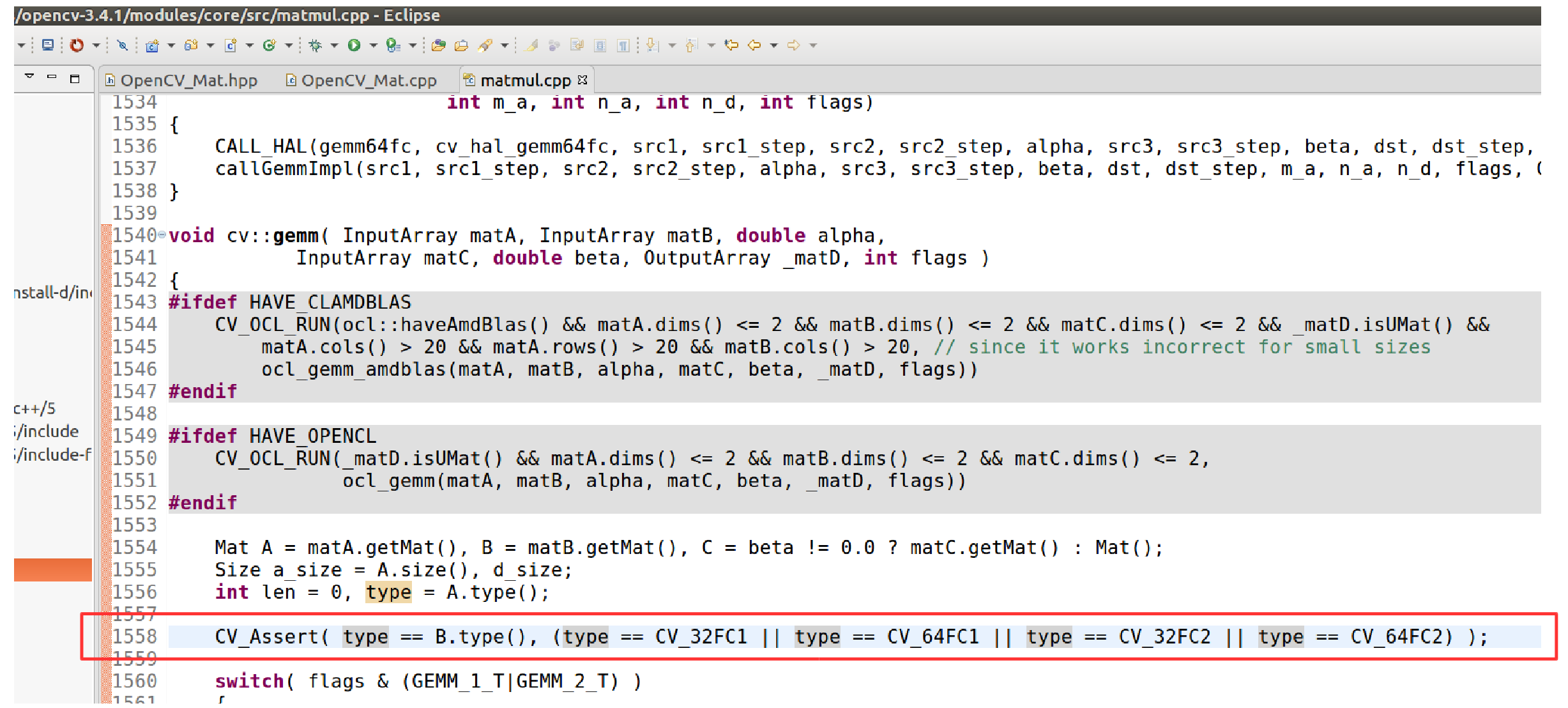# 1 概述

Mat类是OpenCV表达二维图片的基础。

• OpenCV 的API函数，对图像的输出参数都会自动管理内存，调用者可不必亲自管理。但是如果调用者已经对输出参数配置好了合适大小的内纯，API会自动福永该内存而不是重新申请。
• 在使用OpenCV提供的API时，大部分时间不需要考虑内存管理。
• Mat的 = 运算符重载和拷贝构造函数，都不会复制Mat的数据区。若想获得数据区的副本，则需要使用 Mat::clone()和Mat::copyTo()函数。

OpenCV使用BGR格式存储三原色。

Mat的数据格式使用预定义的类似CV_8UC3一样的宏。其中8U代表8bit的无符号整数，C3代表每个pixel有三个通道，8UC3即表示每个pixcel共需要24 bit，3 byte的数据进行存储。注意，在C++代码中，若没有申明using namespace cv; 那么在使用CV_8UC3一类的宏时，不能在前面加 cv:: 作用域修饰，这会导致预编译器在做宏展开时报错

cv::Mat M(2,2, cv::CV_8UC3, cv::Scalar(0,0,255));

OpenCV提供类似于MATLAB的eye(), ones(), zeros()函数，但需要指定数据格式。

Mat的 << 操作符支持使用不同的格式输出，输出的格式涵盖python默认，numpy和c语言的格式。

# 3 算数运算

## 3.3 矩阵乘法

OpenCV强制规定，Mat之间的乘法只能在如下4种数据格式上进行：CV_32FC1, CV_32FC2, CV_64FC1 以及 CF_64FC2。并且两个Mat必须具有相同的数据格式。这个规定体现在OpenCV的源码上，以现在手上的版本（3.4.1）为例，该限制出现在 modules/core/src/matmul.cpp: 1558位置，如图所示。A.convertTo(A, CV_64FC1);

cv::Mat A = ( cv::Mat_<unsigned char>(3, 3) << 1, 2, 3, 4, 5, 6, 7, 8, 9 );

std::cout << "A = " << std::endl << A << std::endl << std::endl;

// Test the t() function.
std::cout << "A.t() = " << std::endl << A.t() << std::endl << std::endl;
std::cout << "After transposition, A = " << std::endl << A << std::endl << std::endl;

// Test the t() function with assignment.
cv::Mat B = A.t();

std::cout << "B = " << std::endl << B << std::endl << std::endl;
B.at<unsigned char>(1,2) = 10;

std::cout << "After assigning value to B." << std::endl;
std::cout << "A = " << std::endl << A << std::endl;
std::cout << "B = " << std::endl << B << std::endl;

// Test arithmetic operations.
cv::Mat C = A.clone();

C += 1;

std::cout << "C += 1 -> " << std::endl << C << std::endl << std::endl;

C -= 1;

std::cout << "C -= 1 -> " << std::endl << C << std::endl << std::endl;

C *= 10;

std::cout << "C *= 10 -> " << std::endl << C << std::endl << std::endl;

C = A.clone();

C += A;

std::cout << "C += A -> " << std::endl << C << std::endl << std::endl;

C -= A;

std::cout << "C -= A -> " << std::endl << C << std::endl << std::endl;

C = A.clone();
C += 250;

std::cout << "C += 250 -> " << std::endl << C << std::endl << std::endl;

C = A.clone();
C -= 250;

std::cout << "C -= 250 -> " << std::endl << C << std::endl << std::endl;

C = A.clone();
C *= 100;

std::cout << "C *= 100 -> " << std::endl << C << std::endl << std::endl;

cv::Mat D;
A.convertTo(D, CV_32FC1); // Only CV_32FC1, CV_32FC2, CV_64FC1 and, CV_64FC2 could be used to do matrix multiplication.

std::cout << "D = " << std::endl << D << std::endl << std::endl;

D *= D;

std::cout << "D *= D -> " << std::endl << D << std::endl << std::endl;

cv::Mat DD = A.clone();
std::cout << "DD.type() = " << DD.type() << std::endl;
std::cout << "DD = " << std::endl << DD << std::endl << std::endl;
DD.convertTo(DD, CV_64FC1);
std::cout << "DD.type() = " << DD.type() << std::endl;
std::cout << "DD = " << std::endl << DD << std::endl << std::endl;

DD *= 100;
std::cout << "DD *= 100 -> " << std::endl << DD << std::endl << std::endl;

DD.convertTo(DD, CV_8UC1);
std::cout << "DD convert to CV_8UC1  -> "<< std::endl << DD << std::endl << std::endl;

DD = A.clone();
DD.convertTo(DD, CV_64FC1);
DD -= 250;
std::cout << "DD convert to CV_64FC1, -= 250 -> " << std::endl
<< DD << std::endl << std::endl;
DD.convertTo(DD, CV_8UC1);
std::cout << "DD convert to CV_8UC1 -> " << std::endl
<< DD << std::endl << std::endl;

cv::Mat E;
//	E = D + A; // This will cause a runtime exception.
//	std::cout << "E.type() = " << E.type() << std::endl << std::endl;

cv::add( A, D, E, cv::Mat(), CV_32SC1 );
std::cout << "E = A + D -> " << E << std::endl << std::endl;
std::cout << "E.type() = " << E.type() << std::endl << std::endl;

B = 250;

std::cout << "B = 250, E = B + D with CV_32SC1 -> " << std::endl << E << std::endl << std::endl;

std::cout << "B = 250, E = B + D with CV_8UC1 -> " << std::endl << E << std::endl << std::endl;©️2019 CSDN 皮肤主题: 大白 设计师: CSDN官方博客